Math resources Geometry Area

Area of a square

# Area of a square

Here you will learn about finding the area of a square, including counting units squares and multiplying the side lengths.

Students will first learn about the area of a square as part of measurement and data in 3 rd grade.

## What is the area of a square?

The area of a square is the amount of space inside the square. It is measured in square units.

For example,

The square units line up with all sides of the square and do not overlap. The area of the square is the number of square units within it.

Notice the connection between the side of a square and the square units:

The side length is always the same as the number of columns and rows of square units, since a square has equal sides.

This is why the area of square formula is \text {side} \times \text {side.}

For example,

Area is always labeled in square units. You can write it out or use the exponent 2 (a small 2 ) to show the units are square.

For example,

square centimeters (\mathrm{cm}^2), square meters (\mathrm{m}^2), square feet (\mathrm{ft}^2), square inches (\mathrm{in}^2).

The area of other polygons is also measured in square units.

For example,

The square units of the rectangle line up with the sides, just like they did for the square. There are 27 square units inside the rectangle, so that is the area.

Notice that this is not true for the other shapes. Some or all of their sides do not line up with the square units. Counting their square units is not as easy. This is why the area of a circle, triangle, hexagon and other shapes is covered in later grades.

### What is the area of a square?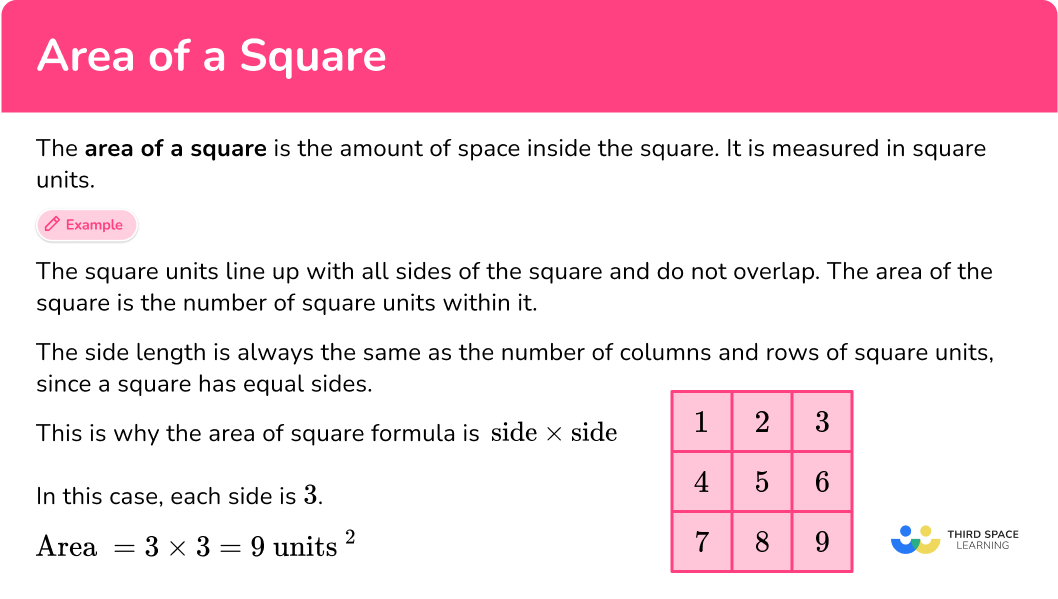## Common Core State Standards

How does this relate to 3 rd grade math and 4 th grade math?

• Grade 3 – Measurement and Data (3.MD.C.5)
Recognize area as an attribute of plane figures and understand concepts of area measurement.
a. A square with side length 1 unit, called “a unit square,” is said to have “one square unit” of area, and can be used to measure area.
b. A plane figure which can be covered without gaps or overlaps by n unit squares is said to have an area of n square units.

• Grade 3 – Measurement and Data (3.MD.C.6)
Measure areas by counting unit squares (square cm, square m, square in, square ft, and improvised units).

• Grade 3 – Measurement and Data (3.MD.C.7a)
Find the area of a rectangle with whole-number side lengths by tiling it, and show that the area is the same as would be found by multiplying the side lengths.

## How to find the area of a square

In order to find the area of a square by counting unit squares:

1. Be sure that the square is made of whole unit squares in an array.
2. Count the unit squares to find the area.

In order to calculate the area of a square:

1. Identify the side length of the square.
2. Write down the formula for the area of a square.
3. Substitute the given values and calculate.

## Area of a square examples

### Example 1: counting unit squares to find the area

What is the area of the square?

1. Be sure that the square is made of whole unit squares in an array.

The unit squares inside the square line up with the sides of the square.

2Count the unit squares to find the area.

The area of the square is 9 square units.

### Example 2: counting unit squares to find the area

What is the area of the square?

The unit squares inside the square line up with the sides of the square.

The area of the square is 36 square units.

### Example 3: counting unit squares (and multiplying the sides) to find the area

What is the area of the square?

Since the length of its sides is 7, the unit squares form a 7 by 7 array.

To find the total number of unit squares (the area) multiply: \text {side} \times \text {side. }

\text { Area }=7 \times 7=49

You can prove this by counting the unit squares within.

The area of the square is 49 square units.

### Example 4: counting unit squares (and multiplying the sides) to find the area

What is the area of the square?

Since the length of each side is 5, the unit squares form a 5 by 5 array.

To find the total number of unit squares (the area) multiply: \text {side} \times \text {side.}

\text { Area }=5 \times 5=25

You can prove this by counting the unit squares within.

The area of the square is 25 square units.

### Example 5: multiplying the sides to calculate the area

What is the area of the square?

Since the length of the sides is the same, all sides are 8 \, cm.

\text { Area of a square }=\text { side } \times \text { side }

\text { Area of a square }=8 \times 8=64

The area of the square is 64 square centimeters.

### Example 6: multiplying the sides to calculate the area

The wall above needs to be painted. How many square feet of paint is needed to cover the wall?

Since the length of a side is the same as the others, all sides are 4 \, ft.

\text { Area of a square }=\text { side } \times \text { side }

\text { Area of a square }=4 \times 4=16

The wall needs 16 square feet of paint to cover it.

### Teaching tips for area of a square

• Make sure students have plenty of practice with tiling squares to find the area (via manipulatives or a digital resource that allows them to do this). These opportunities can provide the conceptual understanding necessary to use the area formula.

• Worksheets can be a great source for practice, but be sure to use worksheets that provide a variety of practice (squares whose area can be found by using physical tiles and squares with or without grids).

### Easy mistakes to make

• Confusing area and perimeter of a square
When first learning about these two concepts, it can be easy to confuse them. Remember that perimeter is the distance around a square and can be found by adding all the sides up (or multiplying one side by 4 ) and area is the space within the square and can be found by multiplying two sides or counting the unit squares within.
For example,

• Not using common units
When tiling a shape to find the area, it is important that all the tiles are the same size. When solving area problems with real world measurements, it is important that all measurements are in the same unit. Having different size tiles or measurements will make the area calculation incorrect.

### Practice area of square questions

1. What is the area of the square?4 square units8 square units12 square units16 square unitsThe unit squares inside the square line up with the sides of the square and form an array.

Count the unit squares to find the area.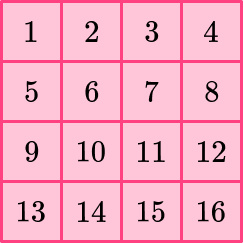The area of the square is 16 square units.

2. Which square has an area of 25 square units?Count the unit squares to find the area.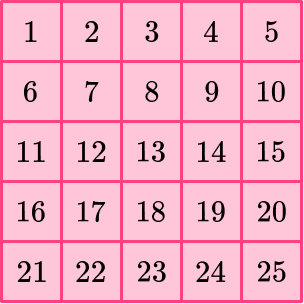The area of the square is 25 square units.

The area can also be found by multiplying \text {side} \times \text {side. } Since 5 \times 5=25, a square with the area of 25 square units will have side lengths of 5.

3. What is the area of the square?6 square units24 square units30 square units36 square unitsSince the length of its sides is 6, the unit squares form a 6 by 6 array.

To find the total number of unit squares (the area) multiply: \text { side} \times \text {side.}

\text { Area }=6 \times 6=36

You can prove this by counting the unit squares within.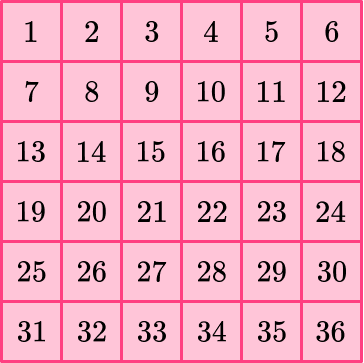The area of the square is 36 square units.

4. What is the area of the square?77 square units64 square units16 square units32 square unitsSince the length of its sides is 8, the unit squares form an 8 by 8 array.

To find the total number of unit squares (the area) multiply: \text { side} \times \text {side.}

\text { Area }=8 \times 8=64

You can prove this by counting the unit squares within.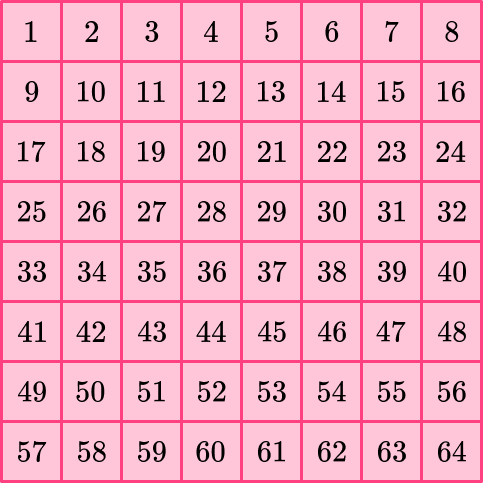The area of the square is 64 square units.

5. What is the area of the square?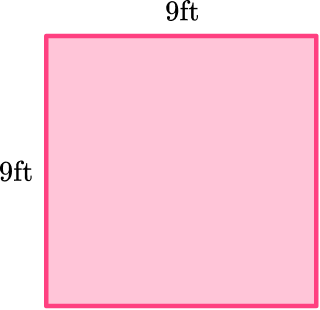81 square feet9 square feet18 square feet36 square feet\begin{aligned}\text { Area of a square } & =\text { side } \times \text { side } \\\\ & =9 \times 9 \\\\ & =81\end{aligned}

The area of the square is 81 square feet.

6. The area of a square is   4 square inches. What is the side length of the square?

1 inch2 inches4 inches16 inches\begin{aligned}\text { Area of a square } &=\text { side } \times \text { side } \\\\ 4 &=\text { side } \times \text { side }\end{aligned}

Think about a number times itself that equals 4…

Since 2 \times 2=4, the square has a side length of 2 inches.

## Area of a square FAQs

How do you calculate the area of a square that has fractions?

To calculate the area of a square that has fractional side lengths, you still multiply \text {side} \times \text {side,} so you can either use a calculator or use what you know about multiplying fractions to solve.

How is the area of the square connected to the perimeter of the square?

Both the area and the perimeter are calculated using the side lengths, but they are not calculated in the same way. The formula for perimeter of a square is 4 \times \text {side length} and the formula for area is \text {side length} \times \text {side length.}

What is surface area?

Surface area is the area of the faces of 3D figures. It is found by calculating the area of each side of a shape and then adding them all together.